Trial ends in
JoVE Science Education
Materials Engineering

A subscription to JoVE is required to view this content.
You will only be able to see the first 20 seconds.

Electrochemical Impedance Spectroscopy
Science Education (Materials Engineering)
Hydrogel Synthesis
Science Education (Materials Engineering)
Optical Materialography Part 1: Sample Preparation

Electrochemical Impedance Spectroscopy

Overview

Source: Kara Ingraham, Jared McCutchen, and Taylor D. Sparks, Department of Materials Science and Engineering, The University of Utah, Salt Lake City, UT

Electrical resistance is the ability of an electrical circuit element to resist the flow of electricity. Resistance is defined by Ohm's Law:(Equation 1)

Whereis the voltage andis the current. Ohm's law is useful for determining the resistance of ideal resistors. However, many circuit elements are more complex and can't be described by resistance alone. For example, if an alternating current (AC) is used then the resistivity will often depend on the frequency of the AC signal. Instead of using resistance alone, electrical impedance is a more accurate and generalizable measure of a circuit element's ability to resist the flow of electricity.

Most commonly, the goal of electrical impedance measurements is the deconvolution of a sample's total electrical impedance into contributions from different mechanisms such as resistance, capacitance, or induction.

Principles

During Electrochemical Impedance Spectroscopy (EIS) an AC voltage is applied to a sample at different frequencies and the electrical current is measured. When dealing with AC currents impedance (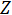) replaces resistance (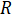) in Ohm's law. If the original AC signal is sinusoidal then a linear response means the current produced will also be sinusoidal, but shifted in phase. Accounting for the frequency and phase shift of the voltage and current is most easily accomplished by utilizing Euler's relationship and complex numbers where we have both a real and an imaginary component to. From this we can build equations for impedance for different components of a circuit:

1.Resistor: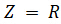(Equation 2)

2.Capacitors:(Equation 3)

3.Inductor: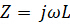(Equation 4)

Whereis the frequency of the AC current,is the capacitance,is the inductance, andis the imaginary unit. From these equations you can see that impedance as a resistor is independent of frequency, inversely related to frequency as a capacitor, and directly related frequency as an inductor.

A Nyquist plot is generated from the frequency response to the electrical impedance by plotting the imaginary component on the y-axis and the real component on the x-axis. The instrument applies an alternating field voltage to the sample and measures the current response. The real and imaginary components of impedance are calculated by determining the phase shift and change in amplitude at different frequencies. An example of this is shown in Figure 1. This plot is then used to build a circuit model that best represents the impedance of the sample.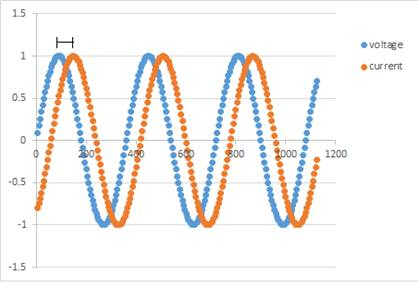Figure 1: A representation of the phase shift between the applied voltage and the measured current.

One of the simplest Nyquist plots is that of a semi-circle which can be seen in Figure 2. The plot in Figure 2 is represented by a resistor in series followed by a resistor and capacitor in parallel-- this is known as equivalent circuit modeling. Different physical processes correspond to elements in the circuit model; for example, an electrical double layer corresponds to a capacitor. In Figure 2, a Nyquist plot is shown that is best modeled by a Randle cell. This a common starting place for the interpretation of a Nyquist plot. After the Nyquist plot is complete, the software will present you with equivalent circuit models you can choose from to model your data. If the Nyquist plot does not have a good fit from the computer-generated fits, you can build your own circuit to fit the data. However, this can be a complicated task. It is important to start simple and build up from there. It is also important to remain realistic based off what you know about the sample you are testing, to ensure that you do not build an unrealistic model. For starters, if the first point is on the real axis, it's commonly modeled as a resistor. As you move along the curve you can add or remove circuit elements to generate a better fit.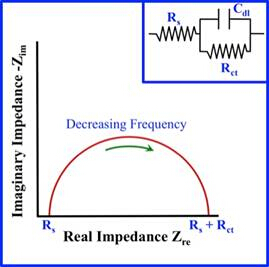Figure 2: Image of a simple Nyquist Plot and its equivalent Randle cell model.

The concept that we plan to model in this experiment is how to test samples with EIS and use the Nyquist plot to build a model circuit which might represent the observed impedance data. For the first part of the experiment we will demonstrate how to run a control sample that produces a known circuit model that the software can easily recognize. For the second part, we can demonstrate how to test an experimental sample and again use the software to generate a model circuit the best models the electrical impedance of the sample.

Procedure

1. Obtain a test module and hook it up to the EIS instruments via two electrodes. The test module, pictured in Figure 3, provides data that can be used to model a simple, known circuit. It can be used to confirm that the wires are hooked up to the machine correctly and that all the machinery parts are functioning.Figure 3: Test module.

1. To start flowing current through the sample, open the Zplot software on the computer. From this software you can set up the parameters for your sample as needed. When running a test on the test module, under "Polarization", set the DC Potential to 0, AC Amplitude to 10 mV, and make sure the drop-down arrow says, "vs. Open Circuit". Under the section "Frequency sweep", set the initial frequency to 1x10^6 Hz, the final frequency to 100 Hz, and interval to 10. Also select "logarithmic" and "Steps/Decade". Then press "okay" to start a new reading.
2. Open the Zview software to view the results. Select z' and z'' to plot. The results will be shown on the negative axis-to show them on the positive axis, multiply by -1. Click "measure" then "sweep" to get the measured z' and z'' values. Compare these measured values to the expected values found on the front of the test module, as seen in Figure 2. If the values match, continue to step 4. If not, check all the wiring and equipment to see that everything is connected and functioning properly.
3. Detach the electrodes from the test module.
4. Prepare the sample; for demonstration we will be using a commercially available beta alumina by putting it in the assembly shown in Figure 4. Insert this assembly into the tube furnace, which is located in the hood fume. This set-up is necessary because EIS tests are usually run by varying amplitude (or voltage) and temperature over a certain time frame. For simplification, we will run this experiment at room temperature only.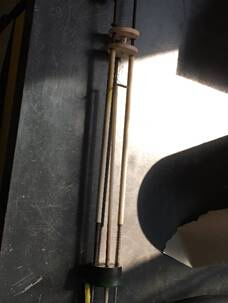Figure 4: Assembly that sample will be inserted into.

1. Attach the electrodes to the assembly as shown in Figure 5.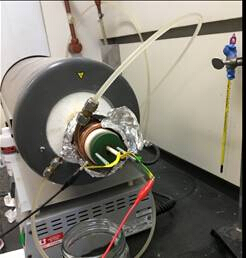Figure 5: Sample assembly, in the hood fume, with electrodes attached.

1. Open the Zplot software and set the parameters. For this experiment, the parameters will be the same as they were in step 2.
2. Obtain the plots using the same procedure as step 3 (except the z' and z'' values do not need to be compared to the test module). Save the plots.
3. Click the "instant fit" button and choose two points to fit the semi-circle. Use the software to choose the best equivalent circuit model.

Electrochemical impedance spectroscopy is a powerful technique used to characterize materials based on how they impede the flow of electricity in applications as diverse as microbiology and corrosion resistance. The electrical conductivity of a sample is based on the make up of all of the components of the sample. Because of this, EIS can also be used to detect changes in the quantity or structure of each component. EIS is performed by applying a small sinusoidal electrical load across electrodes connected to a sample at a wide range of frequencies. Based on the measured response, impedance is computed at each of the frequencies. Computer software is then used to plot the results and build an equivalent circuit model that is representative of the observed data. The typical goal of using EIS is breaking down the sample's total electrical impedance into contributions from mechanisms such as resistance, capacitance, or induction. This video will illustrate the principles and procedures involved in EIS to determine the impedance of a material. It will also demonstrate how to create equivalent circuit models of the sample.

Electrical resistance is the ability of a circuit element to resist the flow of electricity. And Ohm's Law defines resistance as voltage divided by current. When dealing with AC currents, however, electrical impedance is a more accurate and general measure of the ability to resist the flow of electricity. This is because, in addition to the resistance of the material, it accounts for the contribution of mechanisms, such as capacitance and induction. If an applied AC signal is sinusoidal and the response is linear, the current produced will also be sinusoidal, but shifted in phase. To account for the frequency and phase shift we can build impedance equations for components of a circuit using Euler's Relationship and complex numbers. These models are used to interpret data showing impedance to be independent of frequency for resistors, inversely related to frequency for capacitors, and directly related to frequency for inductors. During EIS testing, the instrument applies an alternating field voltage to a sample and measures the current response. The real and imaginary components of impedance are calculated by determining the phase shift and change in amplitude at different frequencies. A Nyquist plot is generated by plotting the imaginary component on the Y axis and the real component on the X axis. One of the simplest Nyquist plots is a semi circle. The plot is then used to build a circuit model that best represents the impedance of the sample. During modeling, physical processes correspond to elements of a circuit. For example, an electrical double layer corresponds to a capacitor. The equivalent circuit model for this plot is represented by a resistor in series with a resistor and capacitor in parallel. This is a common starting place for the interpretation of a Nyquist plot. The software will present you with equivalent circuit models based on your Nyquist plot for you to choose from. If these models do not fit your data you can manually model a circuit to fit the data, a complicated task. In the next section, we will show you how to test a control sample and an experimental sample with EIS and then build an equivalent circuit to represent the observed impedance data.

Gather EIS instruments and a test module. Hook the test module up to the EIS instruments via two electrodes to model a simple known circuit. Open the ZPlot software on the computer to set the parameters for the test module. Set DC potential to zero, AC amplitude to 10 millivolts, and the drop down arrow to versus open circuit. Set initial frequency to one times 10 to power six hertz, final frequency to 100 hertz, and interval to 10. Select logarithmic and steps per decade. Measure, then sweep, to start a new recording, and begin collecting data. Compare measured values to expected values found on the front of the test module. If the values do not match, check wiring and equipment, and retest. Obtain the sample of beta alumina and put it in the assembly. Working in the fume hood, insert the assembly into the tube furnace and attach the electrodes. Open the ZPlot software keeping the same parameters used for the test module and press measure, then sweep. Open the ZView software to view the results as you did for the test module. Save the plots. Choose two points to fit the semi circle. Then press the instant fit button to choose the best equivalent circuit model. For simplification, we ran this experiment at room temperature. EIS tests are usually run by varying amplitude or voltage as well as temperature.

Let's now take a look at our results. Results of the EIS are presented in a Nyquist plot showing real impedance versus complex impedance at each frequency tested. Multiple options of circuits to model your data are provided, it is best to choose the simplest model that still accurately reflects the data. Next, choose an equivalent circuit, and using the resulting data, let's calculate the conductivity of the sample. Data can also be fitted to a linear line using the equation for conductivity. Using the values found through repeated testing for this sample, a conductivity of 1.67 millisiemens per centimeter is calculated, as compared to the reported conductivity value of approximately 4.1 millisiemens per centimeter. This indicates that the model we chose was a good, though not perfect fit.

Now that you appreciate the methods of measuring and modeling impedance using electrochemical impedance spectroscopy, let's take a look at some of the applications for this tool. EIS can be used to look at microorganisms in a sample. When bacteria grow on a sample it can change the electrical conductivity of the sample. Because of this, EIS can be used to measure impedance to determine population growth. This technique is known as impedance microbiology. EIS is also used in the paint and corrosion prevention industries. Materials that show an electrical resistance of less than 10 to the power six Ohms per centimeter square may not protect against the electrical chemical processes that attack surfaces everyday. EIS testing predicts the corrosion resistance properties of materials to be used in harsh environments, saving billions of dollars in repairs every year in the United States alone.

You've just watched JoVE's introduction to electrochemical impedance spectroscopy. You should now understand how to test and model the impedance characteristics of materials. Thanks for watching.

Results

Results of EIS are often presented in a Nyquist plot, which shows real impedance versus complex impedance at each frequency tested. The plot of the experiment ran can be seen in Figure 6.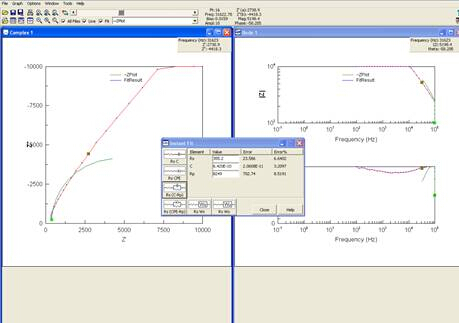Figure 6: Screenshot of computer after Nyquist plot was obtained.

As seen in step 9 of the procedure, the software will give you options of circuits to model your data. It is best to choose the simplest model that still accurately reflects the data. Choosing the correct circuit to model the data is a difficult, inverse problem. While software packages exist that can assist in generating model circuits, care should be taken during this analysis.

When an equivalent circuit is chosen, the resulting data can be used to calculate the conductivity of the sample. One way to calculate conductivity is to plot the data from EIS using an Arrhenius model, which plots 1000/T on the x-axis and log(σT) on the y-axis. The data can be fitted to a linear line using the following equation: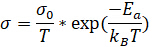(Equation 5)

Where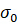for our sample was 374 S/cm*K and Ea, the activation energy, was 0.17 eV, and T = 298 K. Plugging in these values, we calculated a conductivity of 1.67 x 10-3 S/cm. Previous experiments with this sample reported its conductivity to be approximately 4.1 x 10-3 S/cm. This is fairly similar to the conductivity value we calculated, indicating that the model we chose was a good, though not perfect, fit.

Applications and Summary

Electrochemical Impedance Spectroscopy is a useful tool for determining how a new material or device impedes the flow of electricity. It does this by applying an AC signal through the electrodes connected to the sample. The data is collected and plotted by the computer in the complex plain. With the help of software, the graph can be modeled after specific parts of a circuit. This data can often be very complicated and requires careful analysis. This technique, however complex, is an extremely useful non-destructive means of interrogating the real world complexities of electrical impedance, and can provide useful models of how AC current behaves when applied to the sample.

EIS can be used to look at microorganisms in a sample. When bacteria grow on a sample, it can change the electrical conductivity of the sample. Using this idea, you can measure the impedance of a sample at one frequency to determine the population of microorganisms. This technique is known as impedance microbiology.

EIS can also be used to screen for cancer in tissues, known as Tissue Electrical Impedance. The electrical impedance of bodily tissue is determined by its structure. As it degrades over time, it's impedance of electrical current also changes. Much like impedance microbiology, this type of impedance testing looks at the population of cells and can provide useful information about cell heath and morphology.

EIS is also used in the paint and corrosion prevention industries to determine how well a layer is applied to the surface of a material. EIS data corresponds well to every day electrochemical processes that attack surfaces; materials that show an electrical resistance of less than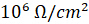may not protect against corrosion as well as materials with a higher resistance. EIS is an avenue to predict how new surface treatments will fair in harsh environments without having to recreate them, making it an invaluable tool in the prevention of corrosion which would otherwise cost the United States billions of dollars in repairs every year.X